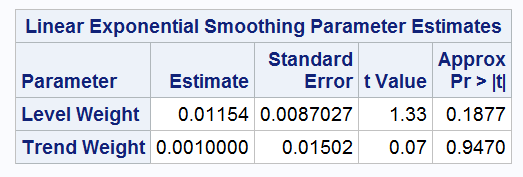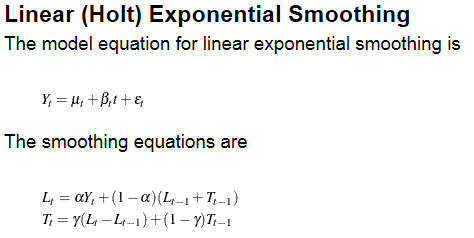## Linear exponential smoothing with PROC ESM

I would like to know how to interpret the parameter estimates in PROC ESM when Holt's exponential smoothing method is used.

``````proc esm data=t print=all ;
forecast x /model=linear;
run;``````

The estimate for Trend Weight is always 0.001.i applied to various real data sets and the simulated data sets with different parameter settings.

``````data t;
a=0.5;
b=0.1;
do t=0 to 100;
x=a+b*t+rannor(123)*0.1;
output;
end;
run;``````

how can they be converted to the parameters, alpha and gamma, specified in the document?thanks.

## Re: Linear exponential smoothing with PROC ESM

Level weight is alpha and Trend weight is gamma.

it just happens that the all data sets i used have constant trends (T(t) = T(t-1)).

the simulation data, of course, have constant trend.

therefore, gamma=0.001 is essentially zero.

Discussion stats